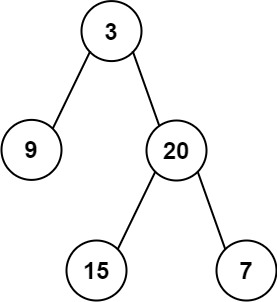# Construct Binary Tree from Preorder and Inorder Traversal

## 1: U-nderstand

Understand what the interviewer is asking for by using test cases and questions about the problem.

• Established a set (2-3) of test cases to verify their own solution later.
• Established a set (1-2) of edge cases to verify their solution handles complexities.
• Have fully understood the problem and have no clarifying questions.
• Have you verified any Time/Space Constraints for this problem?
• Can I expect to receive an empty Array as input?
• No, there may be at least one item in the array
• What is the time and space constraints for this problem?
• `O(nlogn)` time complexity and `O(n)` space complexity
``````HAPPY CASE
Input: preorder = [3,9,20,15,7], inorder = [9,3,15,20,7]
Output: [3,9,20,null,null,15,7]````````````Input: preorder = , inorder = 
Output: 

EDGE CASE
Input: preorder = [-1], inorder = [-1]
Output: [-1]``````

## 2: M-atch

Match what this problem looks like to known categories of problems, e.g. Linked List or Dynamic Programming, and strategies or patterns in those categories.

If you are dealing with Binary Trees some common techniques you can employ to help you solve the problem:

• Think about appropriate Tree Traversal: Pre-Order, In-Order, Post-Order, Level-Order
• Since are traversing a binary tree. We should think about the order, and the order given is Pre-Order and In-Order
• Store nodes within a HashMap to refer to later
• We will need access to the inorder index as determined by value to split the left and right nodes. This will help us improve out time complexity.
• Using Binary Search to find an element
• We are not looking to find an element in this problem so this technique is not useful for this problem
• Applying a level-order traversal with a queue
• Since are traversing tree with the idea of returning a tree using Pre-Order and In-Order values. Level Order Tree Traversal does not apply very well here.

## 3: P-lan

Plan the solution with appropriate visualizations and pseudocode.

General Idea: Pre-Order traverse is process-left-right and In-Order traverse is left-process-right representing a binary search tree. Let's progress and build the tree using the pre-order traversal and ensure the location of the node whether left child or right child by splitting the in-order traverse in half at that value.

``````1. Recursively build Node if Node is in this half of the inorder array
a. Basecase, if inorder array does not exist.
b. Take the first item from preorder to reflect pre-order traversal
c. Find index of root node within in-order traversal to split between left child and right child
d. Recursively build left and right child
2. Return root``````

⚠️ Common Mistakes

• Not seeing the connection between preorder traversal and inorder traversal
• Look at the location of the root node from preorder traversal and inorder traversal and each node thereafter.

## 4: I-mplement

Implement the code to solve the algorithm.

``````# Definition for a binary tree node.
# class TreeNode:
#     def __init__(self, val=0, left=None, right=None):
#         self.val = val
#         self.left = left
#         self.right = right
class Solution:
# Recursively build Node if Node is in this half of the inorder array
def buildTree(self, preorder: List[int], inorder: List[int]) -> Optional[TreeNode]:
# Basecase, if inorder array does not exist.
if not inorder:
return None

# Take the first item from preorder to reflect pre-order traversal
rootVal = preorder.pop(0)
root = TreeNode(rootVal)

# Find index of root node within in-order traversal to split between left child and right child
index = inorder.index(rootVal)

# Recursively build left and right child
root.left = self.buildTree(preorder, inorder[:index])
root.right = self.buildTree(preorder, inorder[index+1:])

# Return root
return root``````
``````public class Solution {
public TreeNode buildTree(int[] preorder, int[] inorder) {
// Basecase, if inorder array does not exist.
if (preorder.length == 0) return null;

// Return helper function
return buildTree(preorder, inorder, 0, 0, inorder.length - 1);
}

private TreeNode buildTree(int[] preorder, int[] inorder, int preorderIndex, int start, int end) {
// Basecase, l and r index crosses
if (start > end) return null;
// Take the first item from preorder to reflect pre-order traversal
TreeNode node = new TreeNode(preorder[preorderIndex]);

// Find index of root node within in-order traversal to split between left child and right child
int inorderIndex = findInorderIndex(inorder, start, end, preorder[preorderIndex]);
int leftTreeSize = inorderIndex - start;
int rightTreeSize = end - inorderIndex;

// Recursively build left and right child
node.left = buildTree(preorder, inorder, preorderIndex + 1, start, inorderIndex - 1);
node.right = buildTree(preorder, inorder, preorderIndex + leftTreeSize + 1, inorderIndex + 1, end);

// Return root
return node;
}

// index finder helper function
private int findInorderIndex(int[] inorder, int start, int end, int key) {
for (int i = start; i <= end; i++) {
if (inorder[i] == key) return i;
}
return -1;
}
}``````

## 5: R-eview

Review the code by running specific example(s) and recording values (watchlist) of your code's variables along the way.

• Trace through your code with an input to check for the expected output
• Catch possible edge cases and off-by-one errors

## 6: E-valuate

Evaluate the performance of your algorithm and state any strong/weak or future potential work.

Assume `N` represents the number of nodes in Tree

• Time Complexity: `O(NlogN)` because we need to visit each node in array. Getting value indexed in inorder would take `O(NlogN)` because we are splitting the inorder array in half with each search, but we still need to make `N` searches. A way to improve this to `O(N)` would be to create a hashmap with the value as key and index as value.
• Space Complexity: `O(N)` because we need to create a resulting tree with the values from array.